# Mathematica入门习题–分段函数的定义

• A+

## 第一题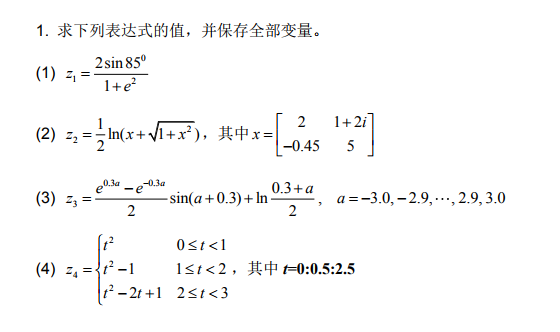### 第一问

``(2 Sin[85 \[Degree]])/(1 + E^2) // N``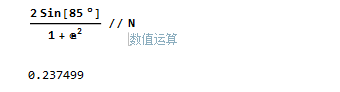### 第二问

``````x = {{2, 1 + 2 I}, {-.45, 5}};
MatrixForm[x]
1/2*Log[x + Sqrt[1 + x^2]] // MatrixForm``````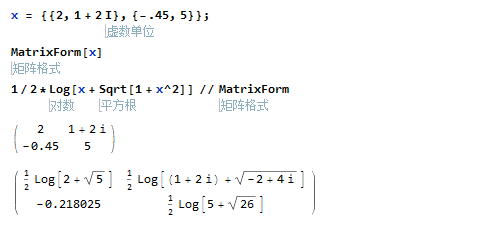### 第三问

``````Table[Log[1/2 (0.3` + a)] +
0.5` (-E^(-0.3` a) + E^(0.3` a)) Sin[0.3` + a], {a, -3, 3, .1}]``````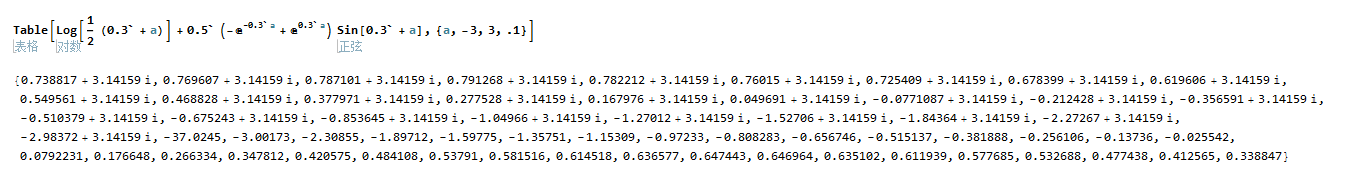### 第四问

``````y[t_] := t^2 /; 0 <= t < 1;
y[t_] := t^2 - 1 /; 1 <= t < 2;
y[t_] := t^2 - 2 t + 1 /; 2 <= t < 3;
Table[y[x], {x, 0, 2.5, .5}]``````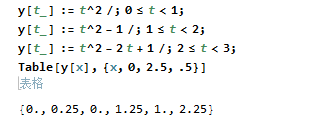• 微信公众号
• 关注微信公众号
•• QQ群
• 我们的QQ群号
•